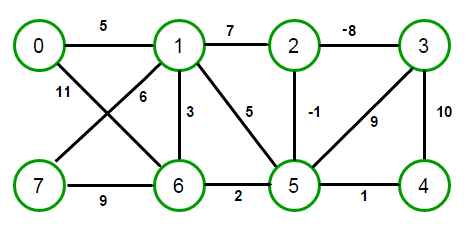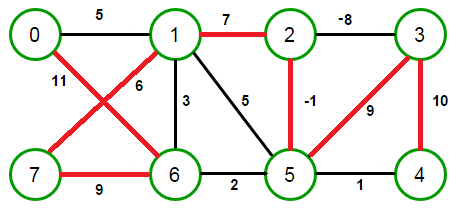# Find maximum cost path in graph from given source to destination

Given a weighted graph, find the maximum cost path from given source to destination that is greater than a given integer x. The path should not contain any cycles.

For example, consider below graph,Let source = 0, k = 40

The maximum cost route from source vertex 0 is 0-6-7-1-2-5-3-4 having cost 51 which is more than k. The solution should return 51.The idea is to do a breadth-first search traversal. BFS is generally used to find shortest paths in graphs/matrix but we can modify normal BFS to meet our requirements. By modifying BFS, we don’t mean using priority queue that picks up the maximum weighted edge at every step as that approach will definitely fail. A low-weight edge can also be involved in maximum cost path as there might be higher weight edges connected through it.

#### So how can we use BFS?

Usually BFS don’t explore already discovered vertex again, but here we do the opposite. In order to cover all possible paths from given source, we remove this check from BFS. But if the graph contains a cycle, removing this check will cause program to go into an infinite loop. We can easily handle that if maintain list of nodes visited so far in current path for a node a queue. Basically we maintain three things in BFS queue node –

• current vertex number
• cost of current path chosen so far
• set of nodes visited so far in current path

So whenever we encounter any node whose cost of path is more, we update the result. The BFS will terminate when we have explored every path that doesn’t result into a cycle.

This is demonstrated below in C++:

Output:

51(1 votes, average: 5.00 out of 5)Loading...

Please use our online compiler to post code in comments. To contribute, get in touch with us.
Like us? Please spread the word and help us grow. Happy coding 🙂Subscribe
Notify ofGuest

Nice oneGuest

This is a classical NP-hard problem.
So, is the solution, like, exponential time, or does it solve P = NP?